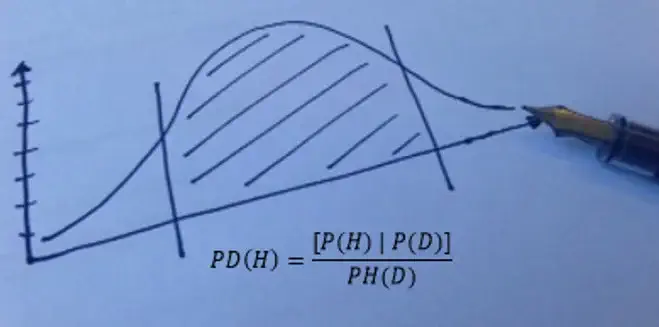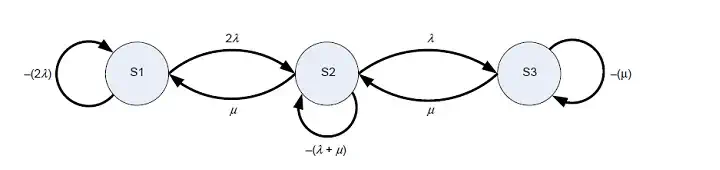## SO 31000 Risk management techniques - continued

### Attributes of a selection of risk assessment tools

There are twelve posts in this series. To read Part IV, please click here.

ISO 9001 Risk-based thinking could (and I am not saying that it should) be demonstrated by one or more of the risk assessment tools in ISO 31010.

Note: the text is based on the contents of Table A.2 – Attributes of a selection of risk assessment tools [Source: IEC/FDIS 31010:2009].

Continuing with ...

## STATISTICAL METHODS

ISO 31010 lists the following statistical methods for risk assessment:

• Markov analysis
• Monte-Carlo analysis
• Bayesian analysis

## Markov analysis

A method named after a Russian mathematician, best known for his work on stochastic processes, where a collection of random variables represents the evolution of some system of random values over time.

Markov analysis, or State-space analysis, is commonly used in the analysis of repairable complex systems that can exist in multiple states, including degraded states1,  and where the use of a reliability block analysis would be inadequate to properly analyse the system.

The nature of the Markov analysis techniques lends itself to the use of software. There are several to choose from on the market.

The Markov analysis process is a quantitative technique and can be discrete (using probabilities of change between the states) or continuous (using rates of change across the states).

To quote ISO 31010:

"The Markov analysis technique is centred around the concept of “states”, e.g. “available” and “failed”, and the transition between these two states over time based on a constant probability of change. A stochastic transitional probability matrix is used to describe the transition between each of the states to allow the calculation of the various outputs."2

The inputs essential to a Markov analysis are as follows:

• list of various states that the system, sub-system or component can be in (e.g. fully operational, partially operation (i.e. a degraded state), failed state, etc);
• a clear understanding of the possible transitions that are necessary to be modelled. For example, failure of a car tyre needs to consider the state of the spare wheel and hence the frequency of inspection;
• rate of change from one state to another, typically represented by either a probability of change between states for discrete events, or failure rate (λ) and/or repair rate (ì) for continuous events.3

The output from a Markov analysis is the various probabilities of being in the various states, and therefore an estimate of the failure probabilities and/or availability, one of the essential components of a system.

#### Strengths and limitations of a Markov analysis

Markov diagrams for large systems are often too large and complicated to be of value in most business contexts and inherently difficult to construct. Markov models are more suited to analysing smaller systems with strong dependencies requiring accurate evaluation. Other techniques, such as Fault Tree analysis (see Part IV of this blog post series), may be used to evaluate large systems using simpler probabilistic calculation techniques.

States depend on current state probabilities and the constant transition rates between states - see the state transition diagram in Figure 1 below:Figure 1: Example of a state transition diagram

Apart from this obvious drawback (complexity), a true Markovian process would only consider constant transition rates, which may not be the case in a real-world systems. Events are statistically independent since future states are treated as independent of all past states, except for the state immediately prior. In this way the Markov model does not need to know about the history of how the state probabilities have evolved in time in order to calculate future state probabilities. However, computer programs are being marketed that allow time-varying transition rates to be defined.

Markov analysis requires knowledge of matrix operations and the results are - unsurprisingly! - hard to communicate with non-technical personnel.

If you would like to perform Markov analysis, you are advised to consult:

## Monte-Carlo analysis

Monte Carlo analysis consists of a broad class of computational algorithms that rely on repeated random sampling to obtain numerical results. This method can address complex situations that would be very difficult to understand and solve by an analytical method. Whenever there is significant uncertainty in a system and you need to make an estimate, forecast or decision, a Monte Carlo simulation could be the answer.

### How does Monte Carlo analysis model the effects of uncertainty?

Systems are sometimes too complex for the effects of uncertainty on them to be modelled using analytical techniques. However, they can be evaluated by considering the inputs as random variables and running a number N of calculations (so-called simulations) by sampling the input in order to obtain N possible outcomes of the wanted result.

Monte-Carlo analysis can be developed using spreadsheets, but software tools are readily available to assist with more complex requirements, many of which are now relatively inexpensive.

Monte-Carlo analysis can be developed using spreadsheets, but software tools are readily available to assist with more complex requirements, many of which are now relatively inexpensive.

Monte Carlo simulations require you to build a quantitative model of your business activity, plan or process.  This is often done by using Microsoft Excel with a simulation tool plug-in - a relatively inexpensive set of tools.

To deal with uncertainties using Monte Carlo analysis in your model, you'll replace certain fixed numbers -- for example in spreadsheet cells -- with functions that draw random samples from probability distributions.  And to analyze the results of a simulation run, you'll use statistics such as the mean, standard deviation, and percentiles, as well as charts and graphs.4

For risk assessment using the Monte Carlo simulation, triangular distributions or beta distributions are commonly used.

Note that ISO 31010 Table A.1 – Applicability of tools used for risk assessment states this is tool is strongly applicable for the Evaluation stage of risk assessment but not applicable (NA) for risk identification or risk analysis.

## Bayesian analysis

Referring again to Table A.1 from ISO 31010, Bayesian analysis is used in the risk analysis and risk evaluation stages in risk assessment.5

In a nutshell, it is a statistical procedure which utilizes prior distribution data to assess the probability of the result. These are often called conditional probabilities.6

There are many places that explain the mathematics behind Bayes' theorem, including Wikipedia, the Stanford Encyclopedia of Philosophy, and the wonderful blog LessWrong. The definition that explains it best for me comes from the last of these - it is:

"The probability of a hypothesis C given some evidence E equals our initial estimate of the probability times the probability of the evidence given the hypothesis C divided by the sum of the probabilities of the data in all possible hypotheses."

Bayesian inference is used in a wide range of fields from medical diagnosis to checking your inbox for likely spam emails. But is it any good for risk assessment?

Although it can appear to be objective, this is typically not the case. A Bayesian probability is really a person’s degree of belief in a certain event rather than one based upon physical evidence.

Because the Bayesian analysis approach is based upon the subjective interpretation of probability, it provides a ready basis for decision thinking and the development of Bayesian nets (or Belief Nets, belief networks or Bayesian networks).7 The availability of software computing tools and what ISO 31010 terms "intuitive appeal" has led to the widespread adoption of Bayesian nets. However, they can be valuable wherever there is the requirement for finding out about unknown variables by using structural relationships and data.

The inputs are similar to the Monte Carlo analysis above; namely:

• define system variables;
• define causal links between variables;
• specify conditional and prior probabilities;
• add evidence to net;
• perform belief updating;
• extract posterior beliefs.8

Bayesian analysis can provide an easily understood model and the data readily modified to consider correlations and sensitivity of parameters.

This technique could be successfully applied to Quality Management Systems, however, there will be minimum sample size requirements for control charts that measure “non-conformities” (errors), based on the average non-conformity rate in the quality processes being measured.

Lower error rates would therefore require larger sample sizes to make valid inferences because of the properties of the binomial distribution.

Even so, I would be very interested to hear from Quality Managers who have applied Bayesian analysis in this way to predict likely error rates in processes!

Notes:

1  ISO/IEC 31010:2009, Table A.2 - Attributes of a selection of risk assessment tools.
2  Ibid. B.24.4 Process, p.70.
3  Ibid. B.24.3 Input, p.70.
4  Monte Carlo Simulation, web page on Frontline Solvers website
5  ISO/IEC 31010:2009, Table A.1 – Applicability of tools used for risk assessment, p.22.
6  ISO/IEC 31010:2009, p.26

7  ISO/IEC 31010:2009, B.26.1 Overview, p.26.
8  Ibid. B.26.3 Input, p.77.

There are twelve posts in this series. To read Part VI, please click here

This post was written by Michael Shuff.